# SSAT Upper Level Math : Number Concepts and Operations

## Example Questions

### Example Question #101 : Arithmetic

Bill decides to donate 15% of his $4000 and Ted decides to donate 25% of his$8000. What percentage of their combined fortunes do Bill and Ted donate?

23.1%

19.2%

22.3%

21.7%

20%

21.7%

Explanation:

This is a simple problem, so long as you don't forget that Bill and Ted have different amounts of money, so you can't simply average 15% and 25% to get 20%.

Calculate how much each donates:

4000(.15)= 600

8000(.25)= 2000

Now divide the total donation by their total wealth: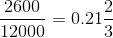Now multiply this answer by 100, and then round to the nearest tenth to get 21.7%.

### Example Question #12 : Arithmetic Word Problems

Joe drove an average of 45 miles per hour along a 60-mile stretch of highway, then an average of 60 miles per hour along a 30-mile stretch of highway. What was his average speed, to the nearest mile per hour?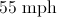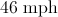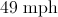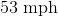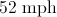Explanation:

At 45 mph, Joe drove 60 miles in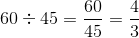hours.

At 60 mph, he drove 30 miles in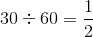hours.

He made the 90-mile trip in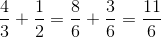hours, so divide 90 by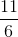to get the average speed in mph: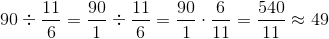### Example Question #1 : Basic Addition, Subtraction, Multiplication And Division

What is the sum of the two percentages in decimal form?

7 percent and 135 percent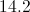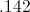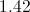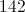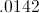Explanation:

You add the percentages and you get 142 percent. To convert a percent to a decimal, you divide by 100, so the answer is 1.42.

### Example Question #1 : Basic Addition, Subtraction, Multiplication And Division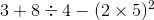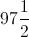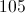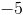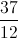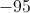Explanation:

Do the expression in the parantheses and you get. Then you do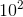and you get. Then you do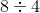and you get. Then you add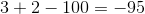.

### Example Question #1 : Basic Addition, Subtraction, Multiplication And Division

If Susie bought a pair of shoes for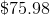, a shirt for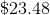, and a pair of pants for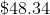. How much did she spend in total?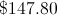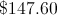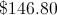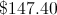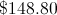Explanation:

You add all three values and you get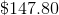.

### Example Question #1 : Basic Addition, Subtraction, Multiplication And Division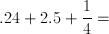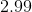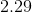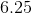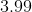Explanation:

First, convert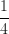into a decimal. We are left with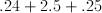Now add the three numbers, being careful to line the decimal points up exactly.  From right to left we will add:

1) 4 and 5 = 9

2) 5, 2, and 2 = 9

3) 2 is alone = 2

This leaves us with 2.99 for the final answer.

### Example Question #1 : Basic Addition, Subtraction, Multiplication And Division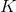andare prime integers: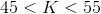and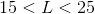. How many possible values of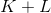are there?

Six

Five

Four

Seven

Eight

Five

Explanation:can be equal to any of the prime numbers between 45 and 55, of which there are two - 47 or 53.can be equal to any of the prime numbers between 15 and 25, of which there are three - 17, 19, and 23.

Their sum can be any of the following: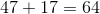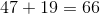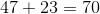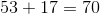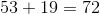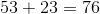This makes five possible sums.

### Example Question #6 : Basic Addition, Subtraction, Multiplication And Divisionandare prime integers.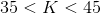and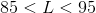. What is the greatest possible value of?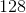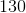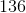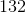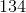Explanation:

To find the greatest possible value of, add the greatest possible value ofto the greatest possible value of. This happens whenis equal to the greatest prime between 35 and 45, which is 43, and whenis equal to the greatest prime between 85 and 95, which is 89. Add the two numbers: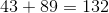### Example Question #1 : Basic Addition, Subtraction, Multiplication And Division

In a math contest, the team from Lincoln High comprised five students - Melanie, Ned, Olivia, Phyllis, and Quinn. Melanie outscored Ned by 12 points; Ned outscored Quinn by 19 points; Phyllis outscored Ned by 45 points and Olivia by 20 points. Who came in third among the five?

Ned

Olivia

Melanie

Quinn

Phyllis

Melanie

Explanation:

Since this reasoning is valid regardless of the actual scores, suppose Quinn scored 100. Then Ned scored 19 more than Quinn, or 119, and Melanie scored 12 more than Ned, or 131. Phyllis scored 45 more than Ned, or 164, and Olivia scored 20 points fewer than Phyllis, or 144. This makes the order, from greatest to least:

Phyllis, Olivia, Melanie, Ned, Quinn

Melanie scored third among the five.

### Example Question #8 : Basic Addition, Subtraction, Multiplication And Division

Which of the following angles is supplementary to an angle that is 45 degrees?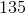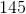Supplementary angles add up to 180 degrees. Given that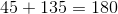, the correct answer is 135.